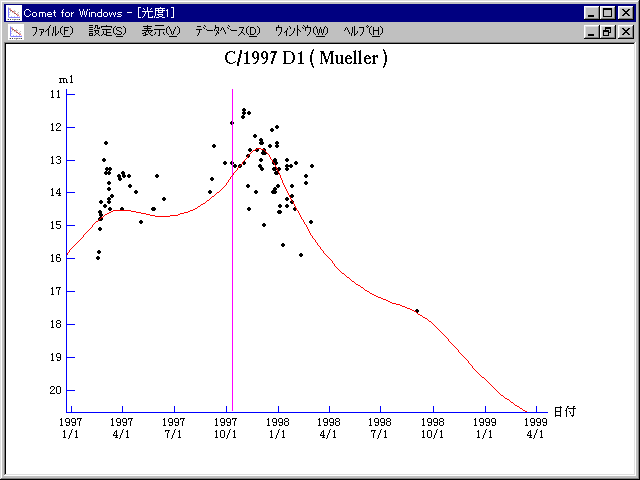# \$B%_%e%i!

C/1997 D1 ( Mueller )###\$B%W%m%U%#!<%k(B

 \$BH/8+F|(B 1997\$BG/(B2\$B7n(B17\$BF|(B \$BH/8+8wEY(B 16\$BEy(B \$BH/8+ Jean Mueller

###\$B50F;MWAG(B

```   The following improved orbital elements by Kenji Muraoka, are
from 421 observations 1997 Feb. 20 to 1998 Sept. 2, perturbations
by 9 Planets, Moon and 5 minor planets were taken into account.
The mean residual is +/- 0.60 arc seconds.

Epoch  =  1997 Sept. 29.0  TT      JDT = 2450720.5
T  =  1997 Oct.  11.61134      +/- 0.00010 (m.e.) TT
Peri. =  184.96032                +/- 0.00003
Node  =  279.17147                +/- 0.00003   (2000.0)
Incl. =  141.88886                +/- 0.00002
q  =    2.2476425              +/- 0.0000005 AU
e  =    1.0011074              +/- 0.0000021
1/a  =   -0.0004927              +/- 0.0000010 1/AU
orig. 1/a  =   +0.000310   1/AU
fut.  1/a  =   -0.000191   1/AU
```

###\$B@1?^(B1997\$BG/(B 1\$B7n(B 2\$BF|!A(B1997\$BG/(B12\$B7n(B 8\$BF|(B1997\$BG/(B12\$B7n(B 8\$BF|!A(B1999\$BG/(B 3\$B7n(B13\$BF|(B

###\$B8wEYJQ2=(B

```        m1 = 10.0 + 5 log\$B&\$(B +  5.0 log r  [ ,0]  (              \$B!A(B1997\$BG/(B10\$B7n(B11\$BF|(B)
m1 =  7.5 + 5 log\$B&\$(B + 12.0 log r  [0, ]  (1997\$BG/(B10\$B7n(B11\$BF|!A(B              )
```##### \$B50F;MWAG\$OB<2,7r<#;a\$N7W;;\$K\$h\$k\$b\$N\$G\$9!#(B \$B@1?^\$O(B StellaNavigator Ver.2.0 for Windows (\$B%"%9%H%m%"!<%D(B \$BJTCx(B / \$B%"%9%-!<=PHG6I4)(B) \$B\$G:n@.\$7\$?\$b\$N\$G\$9!#(B \$B8wEY%0%i%U\$O(BComet for Windows\$B\$G:n@.\$7\$?\$b\$N\$G\$9!#(B